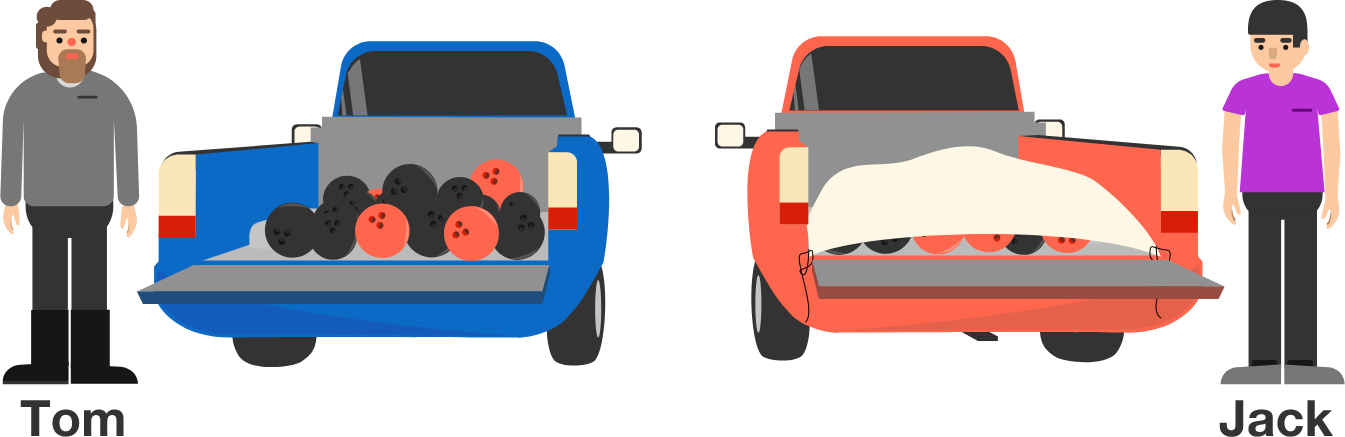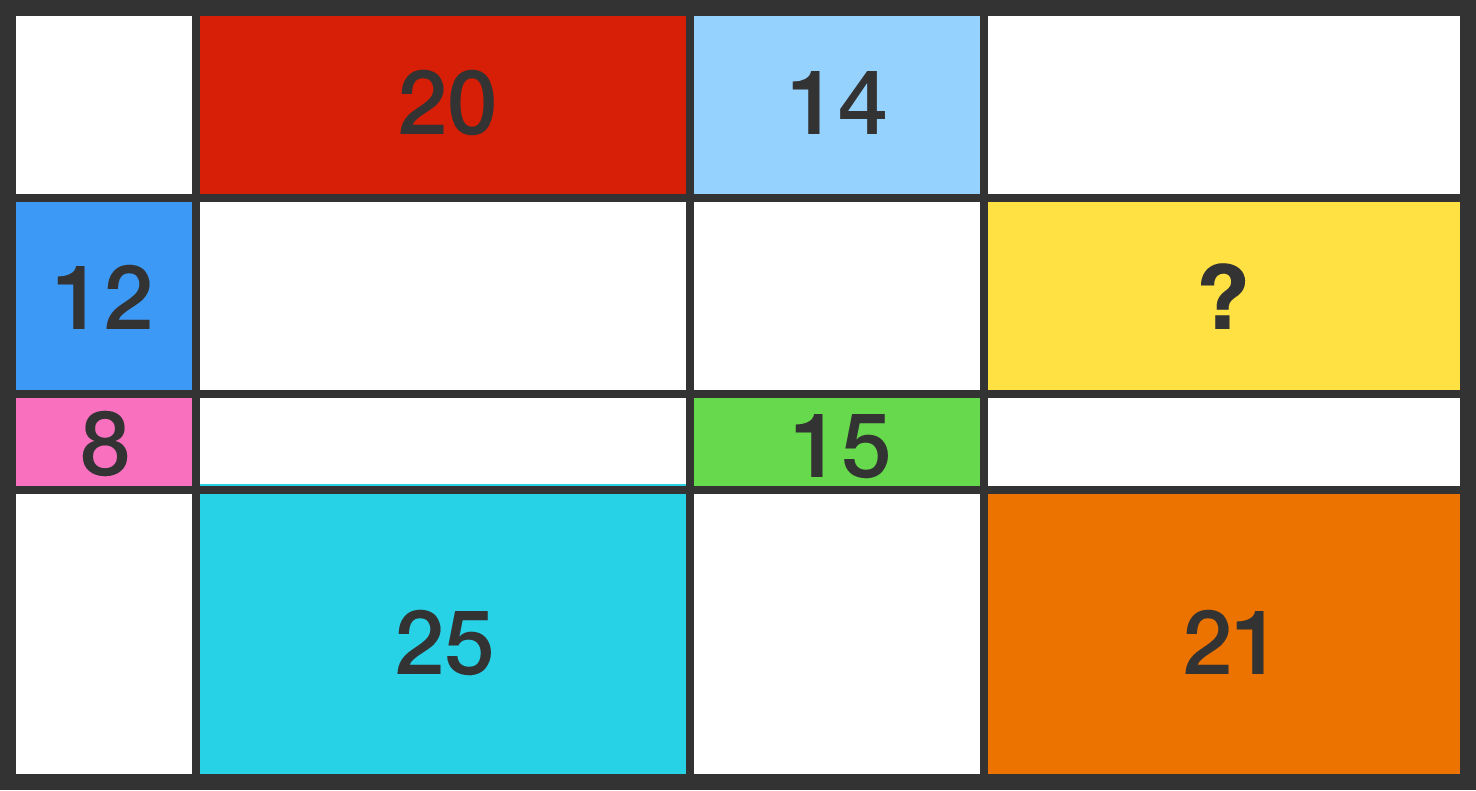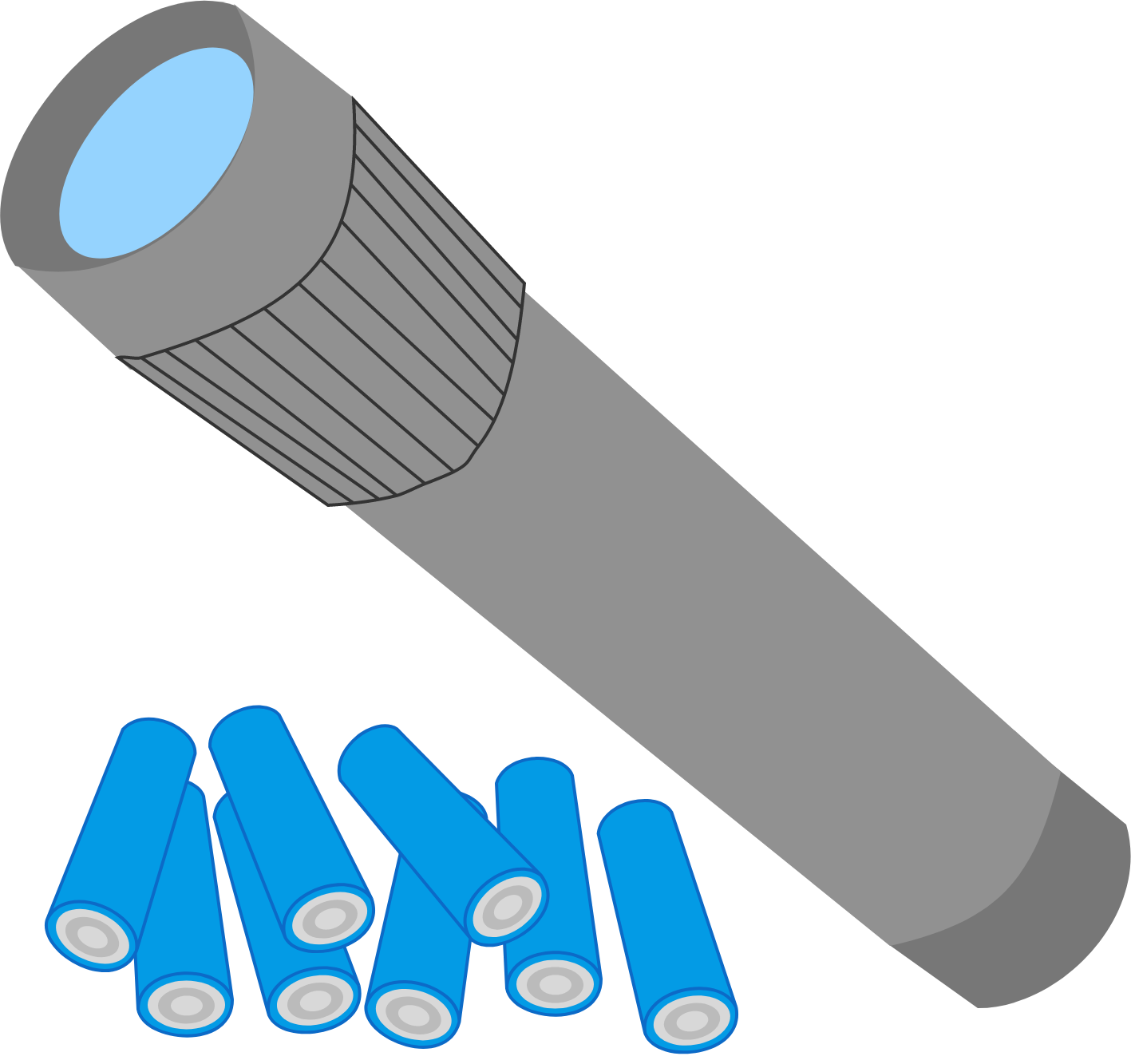# Problems of the Week

Contribute a problem

# 2017-06-26 Intermediate

Tom and Jack each own identical trucks, on which they each carry an equal number of bowling balls with equal weights. Tom keeps the bowling balls loose so they move around on the truck, while Jack has them tightly secured.

If they travel the same route with lots of bumps, pits, and stops, then whose truck will consume less fuel?Details and Assumptions:

• Both the drivers are equally skilled.
• Air drag and other factors that may affect the fuel efficiency of the trucks are also exactly the same for both the trucks.

Celia and Paul are sitting at a round table with a number of other people. They play a game in which they pass a ball around the table, always to the person to the left. The ball starts with Celia, and after 6 passes, Paul has the ball. After 9 additional passes, Celia has the ball again.

Is it possible that Celia and Paul are sitting next to each other?

A rectangle is divided into 16 smaller rectangles, the areas of seven of which are known to us by the numbers in the figure (which is not drawn to scale).What is the area of the yellow rectangle?

A positively charged particle was sitting idle at the origin of a Cartesian coordinate system. The particle is then subjected to an electric field $\textbf{E}=E_{0}\cos\big(\frac{\pi}{2}+\omega t\big)\hat{\imath}$ at time $t\geq0,$ where $E_{0}$ is a positive constant scalar and $\omega$ is the angular frequency of oscillation of electric field in $\text{rad}/\sec.$

What will the particle do after $t=0?$We have 8 batteries: 4 good and 4 dead ones. We don't know which is which, as they all look the same. Now, there is a flashlight which will turn on if it's powered by two good batteries. What is the minimum number of tries we need to guarantee that the flashlight turns on?


Clarification: In a try, we place two batteries into the flashlight and see if it works. The flashlight must turn on with the last try.

×